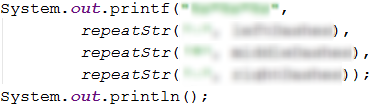# Chapter 6.2. Nested Loops – Exam Problems

In the previous chapter we got familiar with nested loops and to use them drawing different kinds of figures on the console. We learned how to print different size figures, inventing an appropriate logic to construct them using single and nested for loops in combination with various calculations and program logic:

for (int row = 1; row <= 5; row++) {
System.out.print("*");
for (int col = 1; col < 5; col++) {
System.out.print(" *");
}
System.out.println();
}


We also declared our method repeatStr(…) which helps us to print a given symbol (or a series of characters) a certain number of times: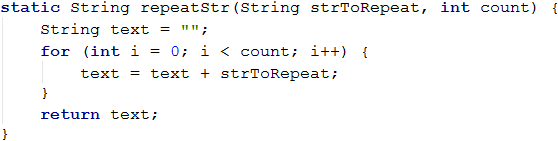## Exam Problems

Let's solve several nested loops related exam problems to practice what we have learned so far and to develop our further algorithmic thinking.

### Problem: Draw a Fort

Write a program that reads from the console an integer n and draws a fortress with width 2 * n columns and height n rows like the examples below. The left and right columns on the inside are n / 2 wide.

### Input Data

The input is an integer n in the range of [3 … 1000].

### Output Data

Print on the console n text rows, depicting the fortress, exactly like the examples.

### Sample Input and Output

Input Output Input Output
3 /^\/^\
|    |
\_/\_/
4 /^^\/^^\
|      |
|      |
\__/\__/
Input Output Input Output
5 /^^\__/^^\
|        |
|        |
|   __   |
\__/  \__/
8 /^^^^\____/^^^^\
|              |
|              |
|              |
|              |
|              |
|     ____     |
\____/    \____/

### Hints and Guidelines

From the problem statement, we see that the input data will be one line only containing an integer within the range [3 … 1000]. Therefore, we will use a variable of type int.Once we have declared and initialized the input data, we need to divide the fort into three parts:

• roof
• body
• base

We can see from the examples that the roof is made of two towers and a middle part. Each tower has a beginning /, a middle part ^, and an end \.\ is a special symbol in Java. Using it in the System.out.println(…) method, the console will not print it. That's why using \\ we instruct the console that we want to print exactly this symbol, without being interpreted as a special one (it is shielded- this is called “character escaping”).

The size of the middle part is n / 2, therefore we can write this value in a new variable. It will store the size of the middle part of the tower.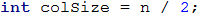Now we declare a second variable, where we will store the value of space between the the two towers. The middle roof part has size of 2 * n - 2 * colSize - 4.To print the roof on the console, we will use our repeatStr(…) method, which accepts two parameters (string, int) and concatenate a certain symbol (or series of characters) n times.The fort body contains a beginning |, a middle part (white space), and an end |. The middle part is a blank space with a size of 2 * n – 2. The number of rows used for walls could be found by the given parameters - n - 3.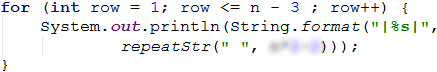To draw the last row, which is a part of the base, we need to print the beginning |, the middle part (white space)_(white space), and an end |. To do this, we can use the declared colSize and midSize variables, because we can see from the examples that they are equal to the _ symbol in the roof.We add to the values of the blank spaces + 1 because we have one blank space more in the examples.

The fort’s base structure is the same as the roof one. It is made of two towers and a middle part. Each tower has a beginning \, a middle part _, and an end /.### Problem: Butterfly

Write a program that reads an integer n from the console and draws a butterfly with 2 * n - 1 columns width and 2 * (n - 2) + 1 rows height as in the examples below. Its left and right sides are n – 1 wide.

### Input Data

The input is an integer n in the range of [3 … 1000].

### Output Data

Print 2 * (n - 2) + 1 text lines, representing the butterfly on the console, just like in the examples.

### Sample Input and Output

Input Output Input Output
3 *\ /*
  @
*/ \*
5 ***\ /***
---\ /---
***\ /***
    @
***/ \***
---/ \---
***/ \***
Input Output
7 *****\ /*****
-----\ /-----
*****\ /*****
-----\ /-----
*****\ /*****
      @
*****/ \*****
-----/ \-----
*****/ \*****
-----/ \-----
*****/ \*****

### Hints and Guidelines

From the problem statement, we see that the input data will be read from only one line, which will contain an integer in the range [3 … 1000]. For this reason, we will use a variable of type int.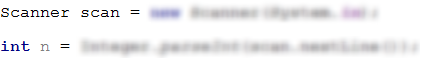We can divide the figure into 3 parts - upper wing, body, and lower wing. To draw the upper wing, we need to divide it into parts - a beginning *, a middle part \ / and an end *. After looking at the examples we can say that the beginning has size n - 2.We also see that the upper wing of the butterfly is of size n - 2, so we can make a loop that iterates halfRowSize times.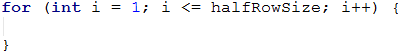From the examples, we can notice that on an even row we have a beginning -, a middle part \ / and an end *, and on an odd line - a beginning *, a middle part \ / and an end -. Therefore, we need to do an if-else condition to check whether the line is even or odd and print one of the two types of lines accordingly.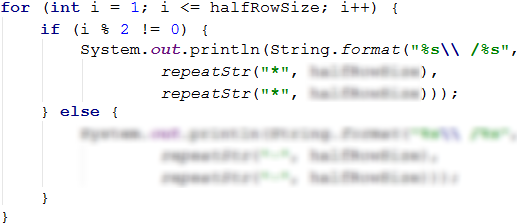To create the body of the butterfly, we can use again the halfRowSize variable and print exactly one line on the console. The body structure has a beginning (white space), a middle @, and an end (white space).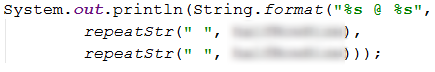It remains to print the lower wing on the console, which is identical to the upper wing.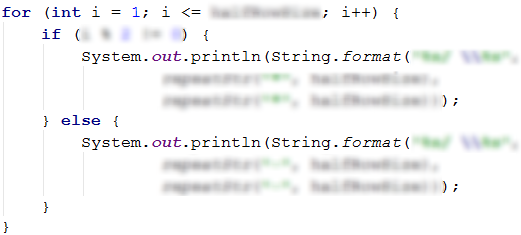### Problem: Stop

Write a program that reads an integer n from the console and draws a STOP warning sign with size as in the example below.

### Input Data

The input is an integer N in the range of [3 … 1000].

### Output Data

Print text lines on the console depicting the STOP warning sign, just as in the examples below.

### Sample Input and Output

Input Output Input Output
3 ...._______....
...//_____\\...
..//_______\\..
.//_________\\.
//___STOP!___\\
\\___________//
.\\_________//.
..\\_______//..
6 ......._____________.......
......//___________\\......
.....//_____________\\.....
....//_______________\\....
...//_________________\\...
..//___________________\\..
.//_____________________\\.
//_________STOP!_________\\
\\_______________________//
.\\_____________________//.
..\\___________________//..
...\\_________________//...
....\\_______________//....
.....\\\___________//.....
Input Output
7 ........_______________........
.......//_____________\\.......
......//_______________\\......
.....//_________________\\.....
....//___________________\\....
...//_____________________\\...
..//_______________________\\..
.//_________________________\\.
//___________STOP!___________\\
\\___________________________//
.\\_________________________//.
..\\_______________________//..
...\\_____________________//...
....\\___________________//....
.....\\_________________//.....
......\\_______________//......

### Hints and Guidelines

From the problem statement, we see that the input data will be read from only one line which contains an integer in the range of [3 … 1000]. Therefore, we will use a variable of type int.We can divide the figure into 3 parts - upper, middle, and lower. The upper part consists of two sub-parts - an initial line and lines in which the character expands. The start row is composed of a beginning ., a middle part _ and an end .. After looking at the examples we can say that the beginning is of size n + 1 and it is better to separate this value into a separate variable.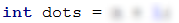We need to create a second variable in which we will store the value of the middle of the initial row and is of size 2 * n + 1.Once we have declared and initialized the two variables, we can print the start line to the console. Let's not forget to pass a new line to the console with System.out.println(…).To draw the lines where the sign is getting "wider", we have to create a loop, that iterates n number of times. The structure of a line consists of a beginning ., // + middle part _ + \\, and an end .. To be able to reuse the created variables, we need to decrease the dots by 1 and the underscores by 2, because we have already printed the first row and the dots and underscores in the next line of the figure decrease.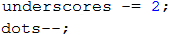At each subsequent iteration the beginning and the end decrease with 1, and the middle part increases by 2.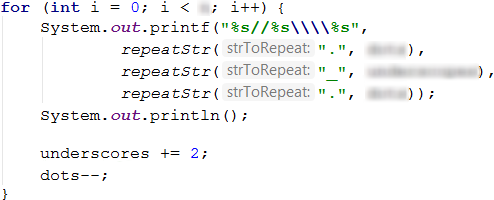The middle part of the figure has a beginning // + _, a middle part STOP! and an end _ + \\. The count of the underscores _ is (underscores - 5) / 2.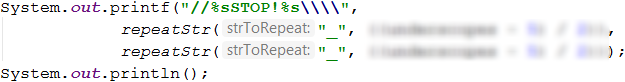The lower part of the figure where the sign decreases can be done by creating a loop again, which iterates n number of times. The structure of a single line is a beginning . + \\, a middle part _ and an end // + .. The number of the dots in the first iteration of the loop should be 0 and increase by one on each subsequent iteration. Therefore, we can say that the size of the dots in the lower part of the figure is equal to i.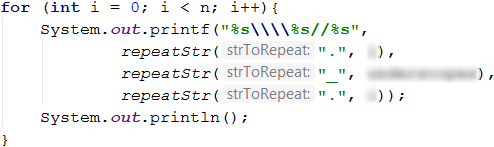For our program to work properly, we need to reduce the number of i by 2 at each iteration of the loop.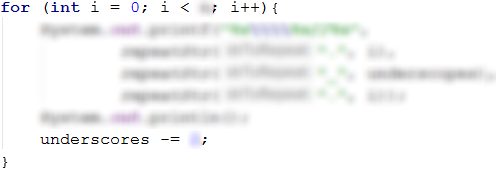### Problem: Arrow

Write a program that reads an odd integer n from the console and draws a vertical arrow pointing down, with dimensions as in the examples below.

### Input Data

The input is an odd integer number n in the range of [3 … 79].

### Output Data

Print a vertical arrow on the console with "#" (hash) outlining the arrow and "." outlining the rest.

### Sample Input and Output

Input Output Input Output
3 .###.
.#.#.
##.##
.#.#.
..#..
5 ..#####..
..#...#..
..#...#..
..#...#..
###...###
.#.....#.
..#...#..
...#.#...
....#....
Input Output
9 ....#########....
....#.......#....
....#.......#....
....#.......#....
....#.......#....
....#.......#....
....#.......#....
....#.......#....
#####.......#####
.#.............#.
..#...........#..
...#.........#...
....#.......#....
.....#.....#.....
......#...#......
.......#.#.......
........#........

### Hints and Guidelines

From the problem condition, we see that the input data will be read from only one line which contains an integer in the range of [3 … 79]. Therefore, we will use a variable of type int.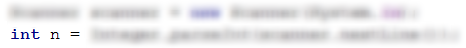We can divide the figure into 3 parts - upper, middle, and lower. The upper part consists of two subparts – the initial row and the arrow body. From the examples, we see that the number of the outer dots in the initial row and in the arrow body are (n - 1) / 2. We can store this value in an outerDots variable.The number of the internal dots in the arrow body is (n - 2). We need to create a variable named innerDots, which will store this value.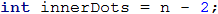We can see from the examples the structure of the initial line. We must use the outerDots and n variables declared and initialized by us to print the starting row. We must remember that we have to move to a new line with System.out.println();.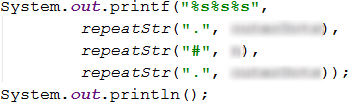To draw on the arrow's body on the console, we have to create a loop, which iterates n - 2 times.The middle part of the figure consists of a beginning #, a middle part . and an end #. We see from the examples that the number of the # is equal to outerDots increased by 1 and that is why we can use the same variable again.To draw the arrow’s lower part, we need to assign new values to the variables outerDots and innerDots.The loop, we are going to make must iterates n - 2times and we need to print the last row of the figure separately. On each iteration outerDots increase by 1, and innerDots decrease by 2.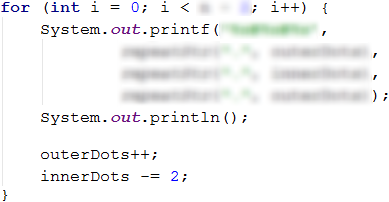The last row of our figure is made of a beginning ., a middle part #, and an end .. The number of the . is equal to outerDots.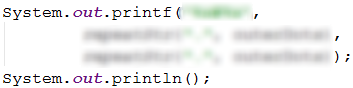### Problem: Axe

Write a program that takes as an input an integer n and draws an axe with size as shown below. The axe is 5 * N columns wide.

### Input Data

The input is an integer n in the range of [2..42].

### Output Data

Print on the console an axe, exactly like the examples.

### Sample Input and Output

Input Output Input Output
2 ------**--
------*-*-
*******-*-
------***-
5 ---------------**--------
---------------*-*-------
---------------*--*------
---------------*---*-----
---------------*----*----
****************----*----
****************----*----
---------------*----*----
--------------********---
Input Output
8 ------------------------**--------------
------------------------*-*-------------
------------------------*--*------------
------------------------*---*-----------
------------------------*----*----------
------------------------*-----*---------
------------------------*------*--------
------------------------*-------*-------
*************************-------*-------
*************************-------*-------
*************************-------*-------
*************************-------*-------
------------------------*-------*-------
-----------------------*---------*------
----------------------*-----------*-----
---------------------***************----

### Hints and Guidelines

To solve the problem, we first need to calculate the dashes on the left, the middle dashes, the dashes on the right, and the whole length of the figure.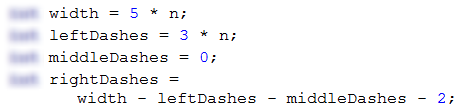Once we have declared and initialized the variables, we can begin drawing the figure by starting with the upper part. From the examples, we can figure out the structure of the first row, and create a loop that iterates n number of times. At each iteration, of the loop the middle dashes increase by 1, and the dashes on the right decrease by 1.To be able to reuse the created variables when drawing the axe’s handle, we need to decrease the middle dashes by 1 and increase the dashes on the right by 1.We can draw the handle of the axe by iterating the loop n - 2 times. From the examples we can understand what its structure is.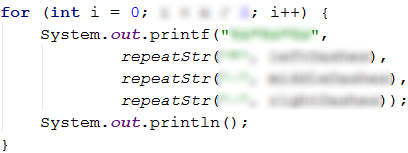The lower part of the figure we have to divide into 2 subparts - the head of the axe and the last row of the figure. We will print the axe’s head on the console by creating a loop that repeats n / 2 - 1 number of times. At each iteration the dashes on the left and the dashes on the right decrease by 1, and the middle dashes increase by 2.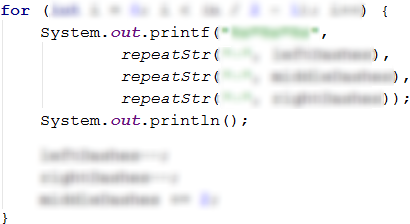For the last row of the figure, we can use the three declared and initialized variables leftDashes, middleDashes, rightDashes again.